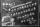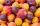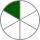# Fraction calculator

The calculator performs basic and advanced operations with fractions, expressions with fractions combined with integers, decimals, and mixed numbers. It also shows detailed step-by-step information about the fraction calculation procedure. Solve problems with two, three, or more fractions and numbers in one expression.

## Result:

### 9/9 - 6/9 = 1/3 ≅ 0.3333333

Spelled result in words is one third.

### How do you solve fractions step by step?

1. Subtract: 9/9 - 6/9 = 9 - 6/9 = 3/9 = 3 · 1/3 · 3 = 1/3
For adding, subtracting, and comparing fractions, it is suitable to adjust both fractions to a common (equal, identical) denominator. The common denominator you can calculate as the least common multiple of both denominators - LCM(9, 9) = 9. In practice, it is enough to find the common denominator (not necessarily the lowest) by multiplying the denominators: 9 × 9 = 81. In the next intermediate step, , cancel by a common factor of 3 gives 1/3.
In words - nine ninths minus six ninths = one third.

#### Rules for expressions with fractions:

Fractions - use the slash “/” between the numerator and denominator, i.e., for five-hundredths, enter 5/100. If you are using mixed numbers, be sure to leave a single space between the whole and fraction part.
The slash separates the numerator (number above a fraction line) and denominator (number below).

Mixed numerals (mixed fractions or mixed numbers) write as non-zero integer separated by one space and fraction i.e., 1 2/3 (having the same sign). An example of a negative mixed fraction: -5 1/2.
Because slash is both signs for fraction line and division, we recommended use colon (:) as the operator of division fractions i.e., 1/2 : 3.

Decimals (decimal numbers) enter with a decimal point . and they are automatically converted to fractions - i.e. 1.45.

The colon : and slash / is the symbol of division. Can be used to divide mixed numbers 1 2/3 : 4 3/8 or can be used for write complex fractions i.e. 1/2 : 1/3.
An asterisk * or × is the symbol for multiplication.
Plus + is addition, minus sign - is subtraction and ()[] is mathematical parentheses.
The exponentiation/power symbol is ^ - for example: (7/8-4/5)^2 = (7/8-4/5)2

#### Examples:

adding fractions: 2/4 + 3/4
subtracting fractions: 2/3 - 1/2
multiplying fractions: 7/8 * 3/9
dividing Fractions: 1/2 : 3/4
exponentiation of fraction: 3/5^3
fractional exponents: 16 ^ 1/2
adding fractions and mixed numbers: 8/5 + 6 2/7
dividing integer and fraction: 5 ÷ 1/2
complex fractions: 5/8 : 2 2/3
decimal to fraction: 0.625
Fraction to Decimal: 1/4
Fraction to Percent: 1/8 %
comparing fractions: 1/4 2/3
multiplying a fraction by a whole number: 6 * 3/4
square root of a fraction: sqrt(1/16)
reducing or simplifying the fraction (simplification) - dividing the numerator and denominator of a fraction by the same non-zero number - equivalent fraction: 4/22
expression with brackets: 1/3 * (1/2 - 3 3/8)
compound fraction: 3/4 of 5/7
fractions multiple: 2/3 of 3/5
divide to find the quotient: 3/5 ÷ 2/3

The calculator follows well-known rules for order of operations. The most common mnemonics for remembering this order of operations are:
PEMDAS - Parentheses, Exponents, Multiplication, Division, Addition, Subtraction.
BEDMAS - Brackets, Exponents, Division, Multiplication, Addition, Subtraction
BODMAS - Brackets, Of or Order, Division, Multiplication, Addition, Subtraction.
GEMDAS - Grouping Symbols - brackets (){}, Exponents, Multiplication, Division, Addition, Subtraction.
Be careful, always do multiplication and division before addition and subtraction. Some operators (+ and -) and (* and /) has the same priority and then must evaluate from left to right.

## Fractions in word problems:

• In fractionsAn ant climbs 2/5 of the pole on the first hour and climbs 1/4 of the pole on the next hour. What part of the pole does the ant climb in two hours?
• Scouts 44/7 of the students in a school are boys. If 3/8 of the boys are scouts, how many scouts are there in a school of 1878 students?
• Unknown numberI think the number - its sixth is 3 smaller than its third.
• InqualitySolve inequality: 3x + 6 > 14
• Unknown number 6Determine x if 1/6 of x is equal to 2/5 of the number 24.
• DividendsThe three friends divided the win by the invested money. Karlos got three-eighths, John 320 permille, and the rest got Martin. Who got the most and which the least?
• HomeworkIn the crate are 18 plums, 27 apricot and 36 nuts. How many pieces of fruit left in the crate when Peter took 8 ninth: 1. nuts 2. apricots 3. fruit 4. drupe
• Equivalent fractionsAre these two fractions equivalent -4/9 and 11/15?
• Unit rateFind unit rate: 6,840 customers in 45 days
• Reciprocal equation 3Solve reciprocal equation: 1/2 + 2/3=1/x
• Tallest and shortestJenn is the tallest on the team. He is 1 1/2 times as tall as the shortest girl whom is 4 1/4 feet tall. How tall is Jebb?
• One sixthHow many sixths are two thirds?
• Class 8.AThree quarters of class 8.A went skiing. Of those who remained at home one third was ill and the remaining six were on math olympic. How many students have class 8.A?